# March 21, 2015

## Playing with High Voltage

Yesterday I dissected a dead microwave oven and took the step-up transformer.  This transformer is used to power the cavity magnetron, a vacuum tube used to generate microwave electromagnetic radiation.  The transformer has an output of around 2,000 VAC.  I was actually hoping for a higher voltage.  The goal was to create a Jacob's Ladder spark gap effect.  The microwave transformer does have enough voltage to generate the effect, but not enough to get it started.  To the two rods must be shorted to initiate the spark.  This produces a single pass by the spark that walks up the ladder.  In addition to not self-starting, a microwave transformer can produce some serious amperage at that high voltage.  The lights dim when it is arcing, and the setup can only run for a few seconds at a time before becoming too hot.
Pictured is an arc walking the ladder.

# March 20, 2015

## Least Squares Curve Fitting, Part 2

In my last article I demonstrated how to get a generalized form that will do least squares curve fitting when the function takes the form: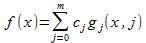Here, c is a set of unknown coefficients, and m is the number of unknown coefficients that are to be solved by the least squares regression. The function g is any function or set of functions that are independent of c. Polynomial regression is a type of least squares regression that can be put into this form, but it is not the only one.

In the past I have written about how there are some non-linear functions that can be linearized and solved in the same manner as polynomial regression. One such function is: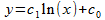This function can be directly translated to our generalized form. We have two coefficients, and it is easiest to write the function g as two functions for this equation: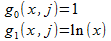Note that j is not used, but this is legitimate—we don't have to use every available variable. Now the generalized form for least squares regression we found yesterday: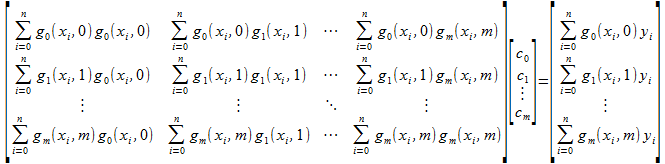Plug in the values for the total number of coefficients: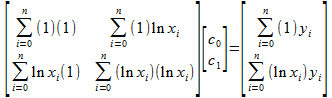Simplify: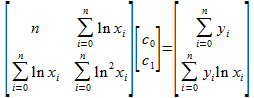And that is the answer we came up with last time.

We can expand on this idea. I don't know if it is useful, but when I implemented this function for the Polynomial Regression library I already had the ability to raise each coefficients term to a power. That is, the Polynomial Regression library has the ability to solve this: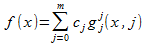Here, the function is raised to the power of the term. What I did for the logarithmic regression was use this function for g: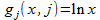And that results in: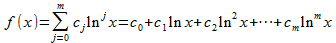The resulting matrix is then: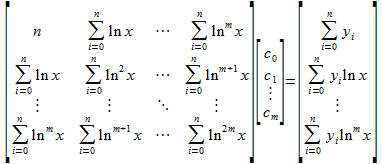Is it useful? I'm not sure, but the structures to make this happen were already in place and easy to do, so why not?

When thinking about other useful forms to place in this function, I thought about physics equations. The one that came to mind first, and is fairly easy to solve, is the derivatives of displacement. We know these as position, velocity, acceleration, jerk, jounce/snap, jaunt/crackle, pop, ext. If one were to take a sample of position at various times, it is possible to use least squares regression to obtain the initial position, velocity, acceleration, ext., assuming they were all constant during the sample period. That means if they have always and continue to stay constant, you can interpolate and extrapolate the position for any point in time.

Let's start with the text-book form of the position equation using just initial position, initial velocity and acceleration: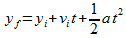This allows one to find the final position (yf) after some amount of time (t) when given an acceleration (a) and initial velocity (vi). Let's rewrite it and change variable names to something inline with what we have been doing so far: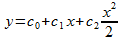Now we can see the coefficients (c) that are the unknowns. Velocity is the change in position over time, and acceleration the change in velocity. We can keep going with this to make a general form for any derivative: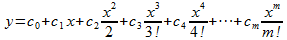With this information we can make a function g to do regression: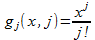Results in: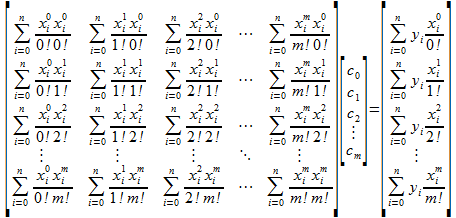Clean this up a bit: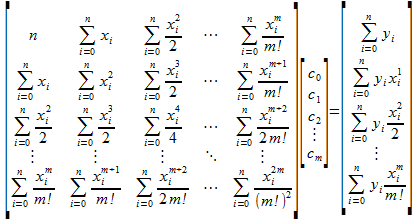Here is an example of the algorithm in action: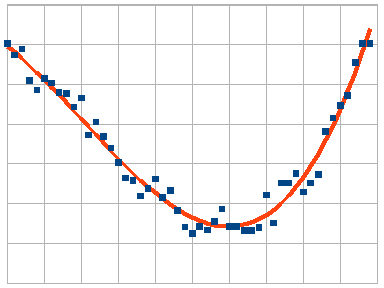Blue dots represent a noisy sample, and the orange line represents the regression curve. The position is positive at the beginning, but because both the velocity and acceleration are initially negative the position decreases. The jerk is positive and reverses this just over halfway through the plot. The initial coefficients (position, velocity, acceleration, and jerk) were (100, -15, -1, 0.1) and the values obtained from the regression were (96.8, -14.6, -1.1, 0.11).

# March 19, 2015

## Least Square Curve Fitting, Part 1

### Math

Polynomial regression is one form of least squares regression. However we can come up with other functions we can solve using least square regression. Here is a non-linear function with a several unknown coefficients: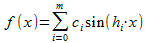Where c is a set of coefficients to be determined, h is a set of known coefficients, and m is the number of coefficients. This function is some kind of amplitude modulator (although I have no idea how useful it might be in any real-world application).

Our function doesn't look linear because it's not. However it is linear with respect to the unknown coefficients c. This is easier to see if we make a substitution: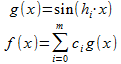The coefficients are independent of the function g. If we ignore whatever is in the sub-function g, the function f is linear. This can be done because the information that is given to the regression analysis is the result of the function (y), and the values for x. Now to do least square regression.

The residual function is a function that specifies the magnitude of error between our true data, and that produced by our estimation function. More specifically, it is the sum of the square of the error, and takes this form: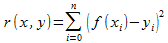Fill this in for our function: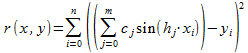Our goal is the minimize this error—to make it as small as possible for a set of x/y data. The residual function is quadratic, which will have one minimum. To find this minimum we need to find where the derivative is zero. Since there are multiple coefficients, we need multiple derivatives—a partial derivative with respect to each coefficient.

This is a little complicated for our equation since most software won't take derivatives in summation form, but we can work around this. So let's expand the inner summation first: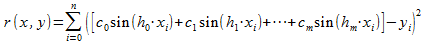We still can't type this into software to take the derivative, but we can use a truncated version to see an emerging pattern. So let's start with the truncated version using just 3 coefficients: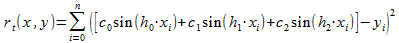Now take the partial derivatives of this: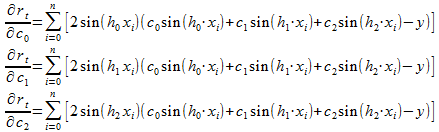There is a pattern emerging: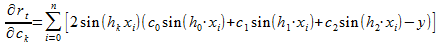Applying this to the non-truncated version of the residual function: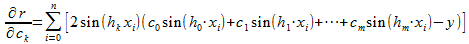And turning that back into a summation: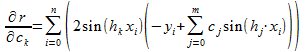The next step is to find where this partial derivative is zero, so set the partial differential to zero. Note that we are just going to do one—there is a partial derivative for each coefficient.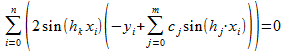Expand: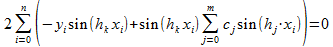Split the summation: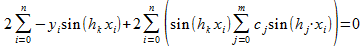Pull out the negative: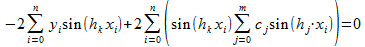Move the y term to the right hand side: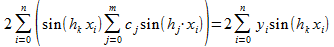Cancel the 2: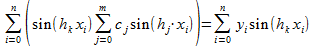In this form it's not obvious how this translates into matrix form. We'll start by expanding the inner summation for each coefficient: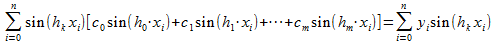Multiply things out: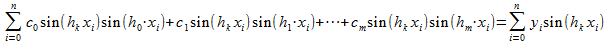Split summation: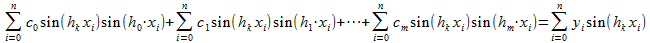Pull out coefficient from summation: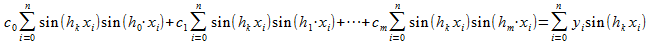Remember that k represents which coefficient of the partial derivative. There are m of these values because there are m coefficients, thus m equations. We get an equation for each coefficient, which expands to: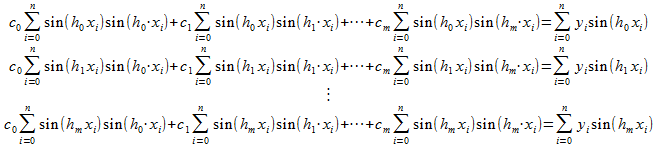And this will translate directly to a matrix: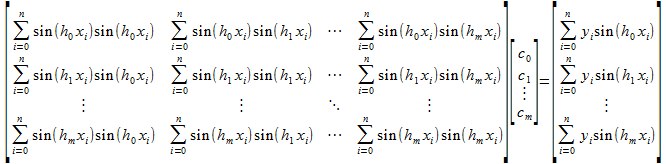There you have it: a least square regression form for our amplitude modulator. Does it work? Here is an example of the function in action: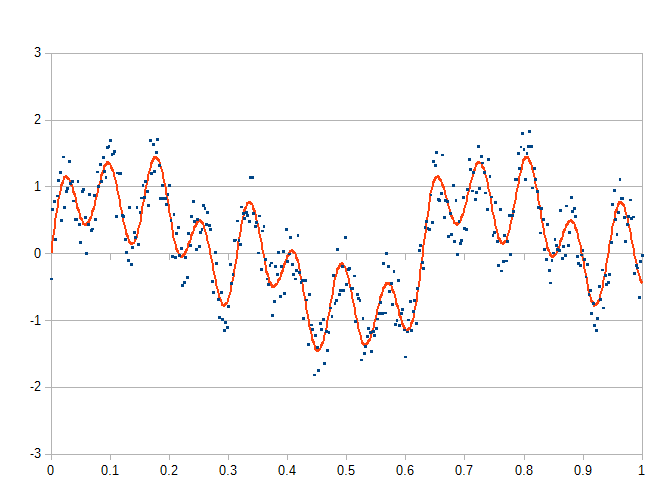The blue dots represent a noisy signal, and the orange line the regression line calculated from the data. The coefficients calculated are pretty close to the originals despite the noise. Again, I don't know that this function is useful, but it does show that least square regression can be done on a function that doesn't look linear.

Let us try and get a more general form. We'll start with the function which consists of some sub-function g that is multiplied by an unknown coefficient. Note I added the subscript j to the function g. The function g could be unique for each coefficient, and this method will still work. In addition, g will get j just in case it wants to use that. The function g must be independent of c (and as it is written will be).The residual function: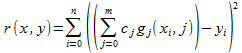Expand inner summation in preparation for partial derivative: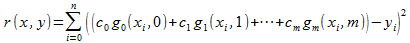Partial derivative: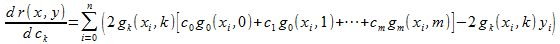Set this equal to zero: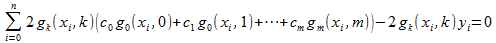Expand: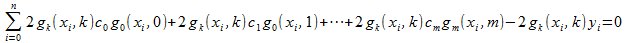Break up summation: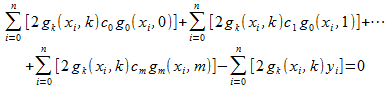Move y terms to right hand side: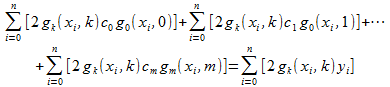Pull constants out of summation: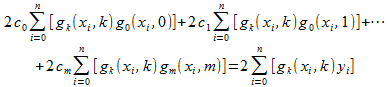Cancel 2: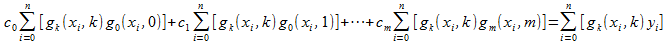Expand to system of equations for each of the m terms: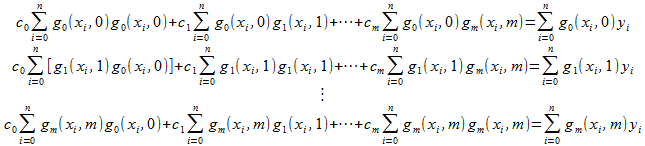And place into matrix form:And this is the general form for least square regression for a linear function. Does it work? Let's try it for polynomial regression. First, express polynomial regression as a function: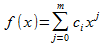Now to put this into the form:For that we need: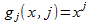Substitute: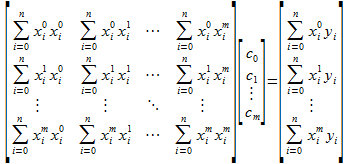Combine like terms and reduce: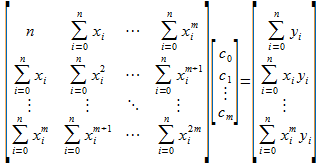And there is the general form of polynomial regression. Thus the general form does work. In subsequent articles we will look at applying the general form to a few other functions.

# March 18, 2015

## Stopping WordPress Brute-Force Bots

After thwarting Bing's efforts to waste my bandwidth, I started looking at rewrite rules to get rid of some of the bots that like to attack my site. I host a couple of WordPress sites, and they seem to be the subject of a number of attacks. Spamming comments and trying to brute force the login seem to be chief among them. I disabled the comment spamming some time ago by removing the script to add comments, but the brute force login I have not done anything with.

As it turns out, attackers don't bother trying to look very authentic when brute forcing a login and password. They make no request for any page but the login script, there is no referring URL, and no user agent. So blocking such requests with a rewrite rule is pretty easy.

RewriteCond %{REQUEST_METHOD} POST
RewriteCond %{HTTP_USER_AGENT} ^\$

Here I fail any request that tries to POST (that is try to login, not request) to the WordPress login script or comments script. It will fail if the referring URL is not from DrQue.net or if the user agent is left blank. A web browser, even if directly linked to the login page, will have to request the page first before doing a POST to login thus satisfying the referring URL. And all real web browsers give a user agent.

In searching through the log file, I found that some robots do have a user agent and referring URL—partly. They don't have it completely accurate. So if it isn't perfect, they can not access the page.

I could have redirected the brute force bot to the ratrap, but I was concerned it wouldn't know how to follow the redirect. The 403 forbidden response will do one of two things. Either they will give up because there is no way to post to a forbidden page, or they will get an endless string of 403 responses as they try. Whatever the case the bot will be thwarted because the login script it is try to brute force is never executed. So even if the bot managed to guess a correct login the it would never know.

# March 17, 2015

## Preventing Bing From Indexing Images

I noticed the other day that Bing (Microsoft's search engine) was ignoring my robots.txt and indexing all the images on DrQue.net. This irritates me a lot. I have over 10,000 images on DrQue.net, and each images exists in 7 sizes. It's a huge waste of bandwidth to index them all, and I don't want them to show up on an image search.  I have no idea why Bing is doing this as all the other real search engines respect robots.txt.  But it wouldn't be the first time Microsoft ignores the standard and does whatever they feel like.  After some searching around, I found other people have had the same problem.

Since I cannot get Bing to respect the conventions, I would have to get a little more drastic.  Somehow I need to prevent the search engine from being able to download images.  The solution is an Apache rewrite rule. The Bing robot at least identifies itself. That being the case, I can ban the user agent from requesting files that match a regular expression.

RewriteEngine on
RewriteCond %{HTTP_USER_AGENT} .*bingbot.* [NC]
RewriteRule ^/Gallery(.*)\$ - [F,L]

After some experimenting this seems to do the trick. The part I was having trouble with is that the rewrite rules must be placed in each virtual host. For some reason I thought it would have to be global, but after making this correction everything worked as expected. What this does is look for a user agent with “bingbot” in the name. If it has that name and the request is for anything in the Gallery directory, a 403 – Forbidden response is returned.  The NC on the RewriteCond makes this non-case sensitive. The [F,L] gives the forbidden response.

I tested this by modifying my brower's header to match the header from the Bing robot. There are several plugins that allow one to modify the user agent, and there are times this can be useful.  Most of the time it is when some antiquated sites (usually government websites) want a specific browser to use their interface.  I've had to do this from Linux machines that require Internet Explorer.  Why would a government website require this?  Because it went to the lowest bidding web monkey who got some kind of Microsoft certificate and now thinks they are qualified to make real websites is my guess.

A few hours after I implemented this I noticed the bing bot getting 403 for image after image. Interestingly, I tried doing an image search on bing for anything from DrQue.net and none of my images showed up. Either this is the first time they have indexed my site and I had not made it into the searches yet, or they take your images but respect the robots.txt enough not to list it. I care not. I was pleased to see 403 after 403 from search.msn.com. It's like thwarting a pesky raccoon.

The blocking works so well, I've decided to change “bingbot” to just “bot”. That should take care of all the major spiders should any of them decide to misbehave.

In addition, I've added several more scam checks to my ratrap redirect.  It's pretty easy to see what scams are out there.  I just look through my log file for what files are often not found.  The ones with huge numbers of hits are usually scams.

# 1 comment has been made.

## From Pluvius

### Platteville, WI

#### March 19, 2015 at 12:16 AM

Nice job - screw Bing and its searching cohorts.

# March 16, 2015

I've decided to start something new today.  Every hour in front of the computer requires 15 push-ups.  I figure if I keep this up, I can continue to increase the number of push-ups.  Initially I had started with 10, but already was able to increase this to 15.  The objective is to make myself move a little more—I spend a lot of time in front a computer screen.
A little afternoon I took my bike out for the first time this year.  Kind of sad I haven't ridden at all before this as the weather has been unseasonably warm.  I got in a quick 5.5 mile ride and did a circle of the Pheasant Branch Trail.  There is a small hill that goes past a water tower on the east side of the trail.  When I started biking last year the hill winded me and made me understand just how out of shape I was.  Despite not having been on my bike since December, I took the hill in a middle gear and got to the top hardly breaking a sweat.  We have only tinny bits of snow left—just hills where it had been piled and are shaded from direct sunlight.  It's still early enough we could get more snow, but late enough that it won't last too long if it does.  I'm always sad to see the snow leave, but at least I'm on my bike again.
In the evening we went swimming.  It has become a bi-weekly event, Monday and Wednesday.  When we started Xen was quite apprehensive about the deep end of the pool, always staying close to the wall.  He got over that and became comfortable in the middle of the deep end.  Then we started a game.  I would drop to the bottom of the deep end, stand on the floor and hold my hand up.  He would dive and try and hit my hand.  The deep end is 12.5 feet deep, and my reach is about 7.5 feet, meaning Xen would have to dive 5 feet to hit my hand.  As he could reach this goal we kept going deeper.  I would kneel on the floor, then sit, and lastly lay on the floor.  The last time we went, I was able to dive all 12.5 feet and grab objects off the floor.  We also got Xen to go off the diving board.  He was apprehensive at first, but now we can't keep him off it.
My own feats swimming have mostly been concentrated on underwater swimming.  The lanes of the pool are 25 meters in length.  Swimming that underwater takes me about 21 seconds and my lungs no longer burn by the time I get to the other side.  I've pool is an L-shape.  There are 8 lap lanes that span 25 meters, and a deep water well for diving.  I don't know how long the well is, but I've been told from one end of the well to the other end of the pool is 25 yards.  Why yards and meters mixed I don't know.  However, my longest swim under water is corner to corner.  Assuming the dimensions are correct, this is a distance of 33.9 meters or 111 feet.  I don't know how long this takes me to traverse, but I can do it completely under water—just barely though.
The other day we tried swimming fins.  I was amazed at how fast I could move with them on.  My normal 25 meter lap takes about 21 seconds.  With fins I was doing it consistently in 16 seconds.  I could drive with so much power from my legs that my arms were pretty much useless.  When I tried to use them they just slowed me down.  I found doing the 33.9 meter corner to corner was only a little easier.  The trick isn't speed—the faster I move the faster I burn the oxygen in my held breath.  The trick is an easy, smooth dolphin kick.  I wanted to try again today, but we didn't have the entire pool.  The last two lap lanes had swimmers doing laps, and I didn't want to get in their way.  So I tried L-shape to the lap lane edge, and then underwater along down the lap lane. I didn't know this distance, but estimate it is around 43 meters (141 feet).  I was able to do this distance under water, but it was difficult.  At rest I can hold my breath for about no more than 120 seconds, but my guess is I cross this distance in under 45 seconds.

# March 15, 2015

## Updates to Online Polynomial Regression Page

Added forcing coefficients to the online polynomial regression page.  This fetcher has been part of the library since last year, but the online interface had no way of utilizing it.  Now any of the coefficients can be forced.  This is mostly used to force an intercept in linear regression, but as I showed back in December of 2013, any coefficient can be forced for any degree polynomial.  With the ability to interact with the regression and force coefficients a user can now refine the coefficients and see the results.  One can force all of the coefficients (at which point no regression analysis is actually done) and see both the graph of the fit as well as the R2 value.  How useful is that?  Well, I am surprised that people comment they have a use for higher degree regression.  So I don't know the answer, but maybe we will get feedback and see.
In addition, I have upped the maximum number of coefficients from 9 to 15.  I had considered upping the maximum amount of data, but the server just isn't fast enough to really handle more than 1,000 data points.  When the Odroid takes over that should change.  In my tests, the Micro-Dragon required 12 seconds to process a 5,000 point data set for a 15 coefficient polynomial.  The Blue-Dragon (which has a proxy of all the sites) requires 2 seconds.
I also added an other example, interpolation.  This example shows how missing data between two sets of data can be filled in with polynomial regression.  It is basically an interactive form of my article from July 19, 2014.  What I like about it is that you can see what varying numbers of coefficients does to the result, and how high numbers of coefficients does not always lead to a better fit.
Other additions include a request for feedback from those who use the page, as well as a warning about the security of doing data analysis with the site.

# March 14, 2015

## Happy Pi Day!

The gathering last night went pretty late, so rather than wait in line for pie at a latter time we decided to get pie for breakfast at 7:30 am.  To celebrate 3/14/15 I have released a new project: the bcextra Arbitrary precision PHP library.  This library was public domain and included in the Gauss-Newton PHP library, but with the addition of the inverse trigonometric I decided to release it under GPL and maintain it.

# March 13, 2015

## Post Apocalyptic St. Patrick's Day Party

The world has ended in a the great poxeclipse.  Just as things began to recover, the dead began to rise and feed upon the living.  Among them, the long dead St. Patrick himself.

# March 12, 2015

## Calculating Inverse Trigonometric Functions To Arbitrary-Precision

### Math

A couple weeks ago I wrote an article about how I failed to apply the Taylor series to the inverse sine function. My goal had been to implement an arbitrary precision inverse sine function and I had found the Maclaurin series for arcsin was converging far too slowly at points near -1 and 1. I had attempted to correct for this by using the Taylor series expanded at -1 and 1 to correct for this, but that was not possible. Since then I have been looking for alternative methods to do this calculation.

The arcsin can be calculated from other inverse trigonometric functions through identities. Most useful of these is the half-angle formula. Using this one can calculate the arcsin using the arctan. That means if I can find a series that converges quickly for inverse tangent, I can also calculate inverse sine and inverse cosine.

Today I found this article on inverse tangent, and it contained two key items. First, a series for inverse tangent that converges faster than the Maclaurin series. And second, a very useful identity. First, the series: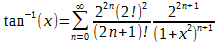The series is credited to “Castellanos 1988” but I haven't found much else about it. Using a spreadsheet I found it does converge significantly faster than the Maclaurin series. Although this series is faster, it still slows down quickly as x increases in magnitude and is best for small values of x. That is where the second identity I found on that page becomes useful.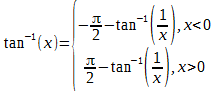This identity allows arctan to use an inverted x which in turn allows one to always use small values for x. If x is too large, just invert it and use the identity. The identity allows x to always stay between -1 and 1.

So I went about making an implementation.

/**
* Computes inverse tangent (arctangent/atan) of x.
*
* @param string \$x Value to take arctangent.
* @return string Arctangent of input.
*/

function bcatan\$x )
{

// Modify the scale for additional accuracy.
// Done after the interval has been reduced to avoid rounds errors with pi.

\$scale bcGetScale();

bcscale\$scale );

if (
bccomp\$x) != )
{

// The series slows down for numbers further from zero.  So for any value
// greater than 1, use this identity:
//                / -pi/2 - atan( 1/x ),       x < -1
//    atan( x ) = | atan( x ),           -1 <= x <= 1
//                \ pi/2 - atan( 1/x ),        x > 1
// This forces the input to always be between -1 and 1, where the series
// converges most quickly.

\$inverse false;
if ( (
bccomp\$x) > )
|| (
bccomp\$x, -) < ) )
{

\$inverse true;

\$x bcdiv1\$x );
}

// Calculate the inverse tangent using Castellanos series for arctan.
//                inf
//               \---  2^(2 n) (n!)^2      x^(2 n + 1)
//   atan( x ) =  >   ---------------- -------------------
//               /---     2 n + 1       (1 + x^2)^(n + 1)
//                n=0

// Couple of constants.

\$xSquared bcmul\$x\$x );

// Loop variables.

\$newResult 0;

\$result = -1// <- Something not equal to \$newResult

\$n 0;

\$factorial    1;

\$oddFactorial 1;

\$xNumerator   \$x;

\$xDenominator \$xFactor;

\$pow2         1;

while (
bccomp\$newResult\$result ) )
{

\$result \$newResult;

\$accumulator bcmul\$factorial\$factorial );

\$accumulator bcmul\$accumulator\$pow2 );

\$accumulator bcmul\$accumulator\$xNumerator );

\$accumulator bcdiv\$accumulator\$oddFactorial );

\$accumulator bcdiv\$accumulator\$xDenominator );

\$n += 1;

\$pow2 bcmul\$pow2);

\$factorial    bcmul\$factorial\$n );

\$oddFactorial bcmul\$oddFactorial\$n );

\$oddFactorial bcmul\$oddFactorial\$n );

\$xNumerator   bcmul\$xNumerator\$xSquared );

\$xDenominator bcmul\$xDenominator\$xFactor );
}

if (
\$inverse )
{

\$accumulator bcdivbcpi(), );
if (
\$x )

\$accumulator bcneg\$accumulator );

\$newResult bcsub\$accumulator\$newResult );
}
}
else

\$newResult NAN;

// Cut off the last few used to account for accumulated error--they are
// incorrect anyway.

\$newResult bcround\$newResult\$scale );

bcscale\$scale );

return
\$newResult;
}

The implementation uses the Castellanos series, but the code is a little tricky because we keep running sums to reduce the total math operations required. For example, rather than calculate (2 n + 1)! each iteration of the loop, the running sum (running product actually) \$oddFactorial is used. After each iteration, it is multiplied by (2 n) and (2 n + 1) which updates the content for the next iteration. This saves a lot of time because n! requires n multiplications per iteration, and this method requires 2.

Now that we have arctan, what about arcsin and arccos? Those are just a simple identity away: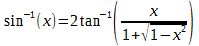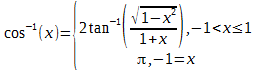So once we have arctan, the other two inverse trigonometric functions are quick to calculate. I have added these functions to my bcextra library. This library is used in my Gauss-Newton PHP Library, but I think I will make a project page just for it—may be useful to someone.

I verified the implementation against 80,000 points between -1 and 1, and 100,000 random points using the built-in inverse trigonometric functions. My calculations (because they are arbitrary precision) are more accurate, so there was naturally an error. This was less than 1e-15, which is about what one can expect for accuracy with conventional floating-point values. I also checked several values against PARI/GP, an open-source computer algebra system. I checked values as much as 250 digits and they matched.